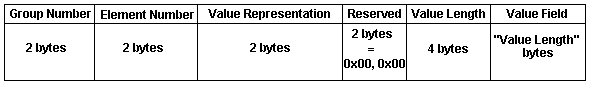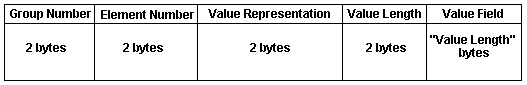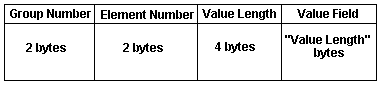Note: This section makes numerous references to VRs such as OB, OW, SQ, etc. For a listing of default information about these Values Representations, refer to the Default Value Representation Table.

## Data Element Structure with Explicit VR

When using the Explicit VR structures, the Data Element shall be constructed of four consecutive fields: Data Element Tag, VR, Value Length, and Value. Depending on the VR of the Data Element, the Data Element will be structured as follows:

• For VRs of OB, OW, SQ and UN, the 16 bits following the two character VR Field are reserved for use by later versions of the DICOM Standard. These reserved bytes shall be set to 0000H and shall not be used or decoded. The Value Length Field is a 32-bit unsigned integer. If the Value Field has an Explicit Length, then the Value Length Field shall contain a value equal to the length (in bytes) of the Value Field. Otherwise, the Value Field has an Undefined Length and a Sequence Delimitation Item marks the end of the Value Field.
• For VRs of UT the 16 bits following the two character VR Field are reserved for use by later versions of the DICOM Standard. These reserved bytes shall be set to 0000H and shall not be used or decoded. The Value Length Field is a 32-bit unsigned integer. The Value Field is required to have an Explicit Length, that is the Value Length Field shall contain a value equal to the length (in bytes) of the Value Field.

Note: VRs of UT may not have an Undefined Length, i.e. a Value Length of FFFFFFFFH.

• For all other VRs the Value Length Field is the 16-bit unsigned integer following the two character VR Field. The value of the Value Length Field shall equal the length of the Value Field.

An example of a Data Element with an Explicit VR, such as would be the case for data type OB, OW, SQ, or UN is shown below:An example of a Data Element with an Explicit VR, such as would be the case for data types other than OB, OW, SQ, or UN is shown below:## Data Element Structure with Implicit VR

When using the Implicit VR structure the Data Element shall be constructed of three consecutive fields: Data Element Tag, Value Length, and Value. If the Value Field has an Explicit Length then the Value Length Field shall contain a value equal to the length (in bytes) of the Value Field. Otherwise, the Value Field has an Undefined Length and a Sequence Delimitation Item marks the end of the Value Field.

An example of a Data Element with an Implicit VR is shown below:Copyright Notices